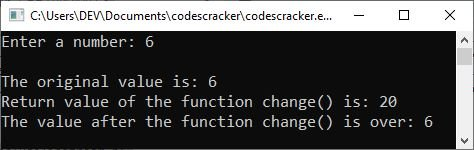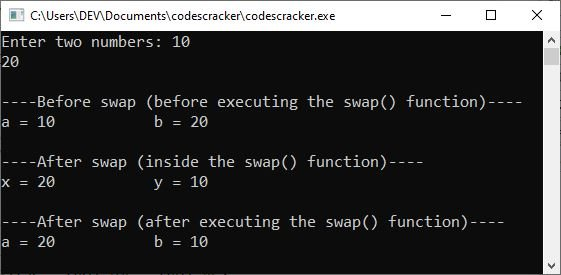# C++ Function Call

A function is called (or invoked, or executed) by providing the function name, followed by the parameters enclosed in parentheses. For instance, to invoke a function whose prototype looks like this:

`float area(float, float);`

The function call statement may look like the one below:

`area(x, y);`

where "x" and "y" have to be float variables. The syntax of the function call is very similar to that of the declaration, except that the type specifiers are missing. Whenever a call statement is encountered, the control (program control) is transferred to the function, the statements in the function body are executed, and then the control returns to the statement following the function call.

## C++ function calling example program

The following program uses function prototyping, function definition, and function calling. This program prints the cube of a given number using a function. This program demonstrates function calling in C++.

```#include<iostream>
using namespace std;

float cube(float);        // function prototype

int main()
{
float num, res;

cout<<"Enter a number to find its cube: ";
cin>>num;

res = cube(num);       // function call

cout<<"\nThe cube of "<<num<<" is "<<res;
cout<<endl;

return 0;
}

float cube(float x)       // function definition
{
float cb;
cb = x*x*x;
return cb;
}```

When the above C++ program is compiled and executed, it will produce the following output:Now type a number, say 5, and hit the ENTER key to find and print its cube, as shown in the snapshot given below:The above program first declares the function "cube" by providing its prototype. Then, after accepting the number, it invokes the function "cube" and assigns the return value of it to the variable "res." As soon as the function call statement is encountered, control gets transferred to the function body, and all its statements are executed. With the execution of a return statement, control returns to the statement immediately following the function call statement.

When a function that expects some arguments is invoked, its call statement must provide the argument values being sent, and the number and type of argument values must be the same as those defined in the function prototype. Also, the order of argument values must be the same as defined in the function prototype. For instance, if a function volume expects two values, the first of float type and the second of int type, then its call statement must look like this:

`volume(a, b);`

where "a" must be a float value and "b" must be an int value.

### How to call a void function in C++

A function that does not expect any arguments is invoked by specifying empty parentheses. For instance, if a function "message" does not expect any arguments, it will be invoked as:

`message();`

Function calls that return values need to have a place in main() for the returned value to reside, to be printed, or to be manipulated. Think of the function as holding out its hand to pass a value back to main(). Thus, main() must stretch out its hand (which may be a variable location) for this value to reside. Let's take another example, demonstrating function calling in C++.

```#include<iostream>
using namespace std;
void welcomeMsg(void);
int findFactorial(int);
int main()
{
welcomeMsg();
int num, fact;
cout<<"Enter a number: ";
cin>>num;
fact = findFactorial(num);
cout<<"\nFactorial of "<<num<<" = "<<fact;
cout<<endl;
return 0;
}
void welcomeMsg(void)
{
cout<<"----Welcome to the program----\n\n";
}
int findFactorial(int x)
{
int res = 1;
for(int i=x; i>=1; i--)
{
res = res*i;
}
return res;
}```

The sample run with user input, 6, is shown in the snapshot given below:## C++ function calling: by value and by reference

A function can be invoked in two ways:

### C++ Call by Value

In the call by value method, the value of the actual parameters is copied into the formal parameters. This means that the function creates its own copy of the argument values, which it then uses.

Please note: When using the call-by-value method, the changes you make will not be reflected back to the values they were originally set to.

The primary advantage of using the call by value method is that it prevents you from modifying the variables that are used to call the function. This is because any change that takes place within the function will have an effect on the copy of the argument value that is used by the function. The original copy of the value being argued for has been preserved in its entirety.

#### C++ function call by value example program

The method of function invocation known as "call by value" will be demonstrated in the following piece of code. The actual parameters to a function call are the ones that are listed in the function call statement. Formal parameters are those that are listed in the function definition itself.

```#include<iostream>
using namespace std;
int change(int);
int main()
{
int orig;
cout<<"Enter a number: ";
cin>>orig;
cout<<"\nThe original value is: "<<orig;
cout<<"\nReturn value of the function change() is: "<<change(orig);
cout<<"\nThe value after the function change() is over: "<<orig;
cout<<endl;
return 0;
}
int change(int a)
{
a = 20;
return a;
}```

The snapshot below depicts a sample run of the above C++ program with user input of 6.In the preceding program, the value of the argument to change(), 6 (entered by the user), is copied onto parameter "a," resulting in a value of 6. When the statement `a = 20;` takes place, the value of "a" is changed but not the value of "orig." The "orig" still has a value of 6.

Please remember: It is a copy of the value of the argument that is passed into the function. The variable used in the function call is not affected by what happens inside the function.

### C++ Call by Reference

The called function, when using the call-by-value method, will first create a new set of variables, and then it will copy the values of the arguments into those variables. The function is only able to operate on the copies of the values that it has created because it does not have access to the original variables, which are the actual parameters. It is helpful to pass arguments by value when the values that were initially passed are not going to be changed. In point of fact, the call by value method provides assurance that the function will not adversely affect the value that was passed to it.

A different mechanism is utilized when the call by reference method is used. A reference to the original variable is sent to the function that is being called rather than the value that was being passed to it from the previous function. Keep in mind that an alias, also known as a different name, for a predefined variable is what we refer to as a reference. That is to say, it is possible to access the value of the same variable by using either of the two names: the name of the original variable, or the reference name.

When you use the call by reference method, any changes you make are reflected back to the values they were in the beginning.

When it is necessary to use a function to alter the values of the original values, it is helpful to use the call by reference method.

#### C++ function call by reference example program

The following program swaps two values using the function call by reference approach in C++.

```#include<iostream>
using namespace std;
void swap(int &, int &);
int main()
{
int a, b;
cout<<"Enter two numbers: ";
cin>>a>>b;
cout<<"\n----Before swap (before executing the swap() function)----\n";
cout<<"a = "<<a<<"\t\t b = "<<b;
swap(a, b);
cout<<"\n\n----After swap (after executing the swap() function)----\n";
cout<<"a = "<<a<<"\t\t b = "<<b;
cout<<endl;
return 0;
}
void swap(int &x, int &y)
{
int temp;
temp = x;
x = y;
y = temp;
cout<<"\n\n----After swap (inside the swap() function)----\n";
cout<<"x = "<<x<<"\t\t y = "<<y;
}```

When the above C++ program is compiled and executed, it will produce the following output with user input of 10 and 20 as two numbers:And if the same program is created using the function call by value approach, or if I remove the "&" before both the variables available in the function prototype and function definition, then here is the output with the same user inputs as done in the previous sample run.

```Enter two numbers: 10
20

----Before swap (before executing the swap() function)----
a = 10           b = 20

----After swap (inside the swap() function)----
x = 20           y = 10

----After swap (after executing the swap() function)----
a = 10           b = 20```

In the above program, the function swap() creates references x for the first incoming integer and y for the second incoming integer. The original values will be used, but with the names x and y.

Note: The & is called the "address of" operator.

C++ Quiz

« Previous Tutorial Next Tutorial »